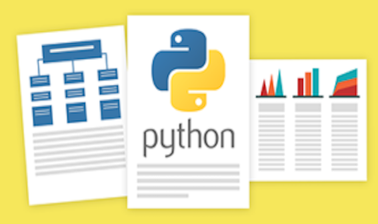# Analyzing Data with Python

In this course, you will learn how to analyze data in Python using multi-dimensional arrays in numpy, manipulate DataFrames in pandas, use SciPy library of mathematical routines, and perform machine learning using scikit-learn!### There is one session available:

120,340 already enrolled! After a course session ends, it will be archived.
Starts Sep 27
Estimated 5 weeks
2–4 hours per week
Self-paced
Free

Please Note: Learners who successfully complete this IBM course can earn a skill badge — a detailed, verifiable and digital credential that profiles the knowledge and skills you’ve acquired in this course. Enroll to learn more, complete the course and claim your badge!

LEARN TO ANALYZE DATA WITH PYTHON

Learn how to analyze data using Python in this introductory course. You will go from understanding the basics of Python to exploring many different types of data through lecture, hands-on labs, and assignments. You will learn how to prepare data for analysis, perform simple statistical analyses, create meaningful data visualizations, predict future trends from data, and more!

### Awards# What you'll learn

Skip What you'll learn
• How to import data sets, clean and prepare data for analysis, summarize data, and build data pipelines
• Use Pandas DataFrames, Numpy multidimensional arrays, and SciPy libraries to work with various datasets
• Load, manipulate, analyze, and visualize datasets with pandas, an open-source library
• Build machine-learning models and make predictions with scikit-learn, another open-source library

It includes following parts:

Data Analysis libraries: will learn to use Pandas DataFrames, Numpy multi-dimentional arrays, and SciPy libraries to work with a various datasets. We will introduce you to pandas, an open-source library, and we will use it to load, manipulate, analyze, and visualize cool datasets. Then we will introduce you to another open-source library, scikit-learn, and we will use some of its machine learning algorithms to build smart models and make cool predictions.

# Syllabus

Skip Syllabus

Module 1 - Importing Datasets

• Learning Objectives
• Understanding the Domain
• Understanding the Dataset
• Python package for data science
• Importing and Exporting Data in Python
• Basic Insights from Datasets

Module 2 - Cleaning and Preparing the Data

• Identify and Handle Missing Values
• Data Formatting
• Data Normalization Sets
• Binning
• Indicator variables

Module 3 - Summarizing the Data Frame

• Descriptive Statistics
• Basic of Grouping
• ANOVA
• Correlation
• More on Correlation

Module 4 - Model Development

• Simple and Multiple Linear Regression
• Model EvaluationUsingVisualization
• Polynomial Regression and Pipelines
• R-squared and MSE for In-Sample Evaluation
• Prediction and Decision Making

Module 5 - Model Evaluation

• Model Evaluation
• Over-fitting, Under-fitting and Model Selection
• Ridge Regression
• Grid Search
• Model Refinement